## Use Of COUNTIF function

In this lesson you can learn about COUNTIF function. COUNTIF function counts the number of times within the specified range of cells that perform the specified value as a criterion.

Function syntax:=COUNTIF (range, criteria)

• Range – the range of cells that you want to count the data. There has to be an ongoing area.
• Criteria – a condition that must be met for cell counting. In the case of numeric values – the condition can look like eg 100

## Count string with exact string of text

You want to count cells where there is only REPORT text. Cells are in C column.

The formula is =COUNTIF(C2:C10,”REPORT”)## Greater than

You want to count cells where value is greater than 10. Cells are in C column.

The formula is =COUNTIF(C2:C10,”>”&10)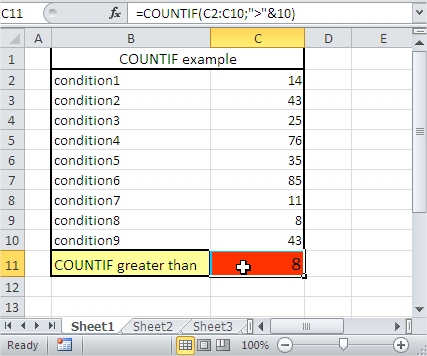## Greater than or equal to

You want to count cells where value is greater than or equal 10. Cells are in C column.

The formula is =COUNTIF(C2:C10,”>=”&10)## Not equal to

You want to count cells where value is not equal to 10. Cells are in C column.

The formula is =COUNTIF(C2:C10,”<>”&10)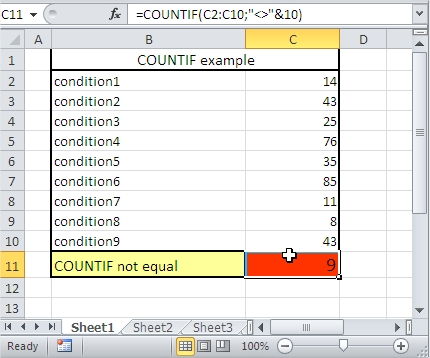## Less than x but more than y

You want to count cells where value is less than 40 but more than 15. Cells are in C column.

The formula is =COUNTIF(C2:C10,”<“&40)-COUNTIF(C2:C10,”<=”&15)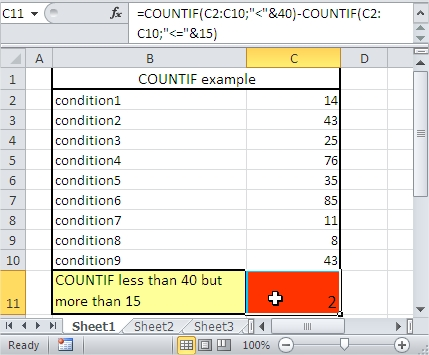## Countif plus countif

You want to count cells where value is greater than 40 plus less than 15. Cells are in C column.

The formula is =COUNTIF(C2:C10,”>”&40)+COUNTIF(C2:C10,”<“&15)## Count cells containing string of text

You want to count cells which contain REPORT text. Cells are in C column. Use asterisk in your formula. Asterisk replaces any other signs.

The formula is =COUNTIF(C2:C10,”*REPORT*”)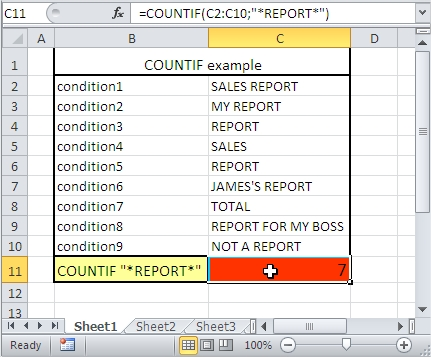## Count cells beginning with string of text

You want to count cells which begin REPORT text. Cells are in C column. Use asterisk in your formula. Asterisk replaces any other signs.

The formula is =COUNTIF(C2:C10,”REPORT*”)## Count cells ending with string of text

You want to count cells which end RT text. Cells are in C column. Use asterisk in your formula. Asterisk replaces any other signs.

The formula is =COUNTIF(C2:C10,”*RT”)## Count cells ending with string of text and contains 6 letters

You want to count cells which end RT text and contains 6 letters. Cells are in C column. Use question mark in your formula. Question mark replaces one sign.

The formula is =COUNTIF(C2:C10,”????RT”)## Count cells containing any text

You want to count cells which contain any text. Cells are in C column. Use asterisk in your formula. Asterisk replaces any other signs.

The formula is =COUNTIF(C2:C10,”*”)## Count cells not containing any text

You want to count cells which not contain any text. Cells are in C column. Use asterisk in your formula. Asterisk replaces any other signs. <> means not equal to.

The formula is =COUNTIF(C2:C10,”<>”&”*”)## Greater than or equal to value from cell

You want to count cells where value is greater than or equal to value from cell C12. Cells are in C column.

The formula is =COUNTIF(C2:C10,”>=”&C12)## Greater than value from cell

You want to count cells where value is greater than value from cell C12. Cells are in C column.

The formula is =COUNTIF(C2:C10,”>”&C12)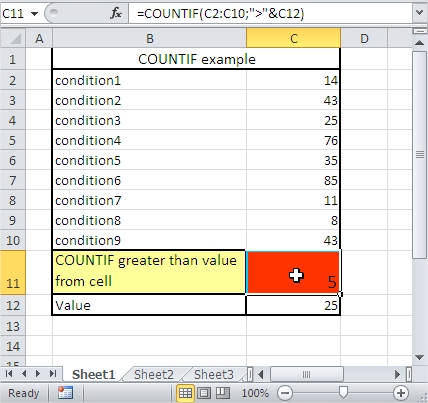Now you know how to use COUNTIF function in Excel. You know also many COUNIF formulas to use in your business cases.

## Template

```Further reading: